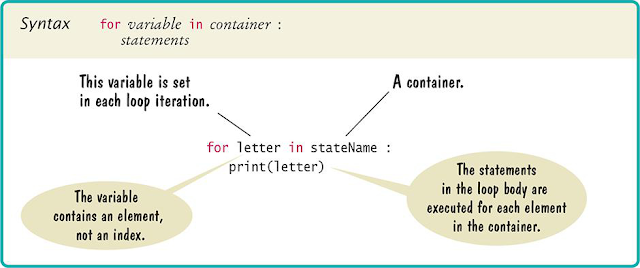### Python For Loop increment Tricky Example

In this post, I have explained tricky for loop example in Python. The usage of FOR LOOP is to create loops. How many times the loop will execute you may supply in the input.My Project is to get each character from the input String. I have used for loop to achieve this. Let me explain how I did this. Explained Tricky FOR LOOP Examples.

#### 1. Python For Loop Array.

>> for value in variable

#### Python For Loop Example.

>> myName = 'srinimf'

>> for value in myName
...        print(value)

The result is as follows:
s
r
i
n
i
m
f

#### 2. Python For Loop With '1' to '10' or 'n'.

```for (i = 1; i <= 10; i++)
<loop body>```
This for loop is useful to create a definite-loop. The maximum number of loops here are '10'. In the body, you need to add Python logic.

This is one of the tricky and most popular examples.

#### 3. Python For Loop a Flow Chart.Explanation:

• The 'letter' contains Value
• The 'stateName' can be an Array or Variable
• After For Loop, you can use BODY statements

Summary
1. The purpose of the for loop is to handle loops
2. You can unstring from the main string
3. You can use for loop with range() built-in function
4. You can handle program logic in the For Loop